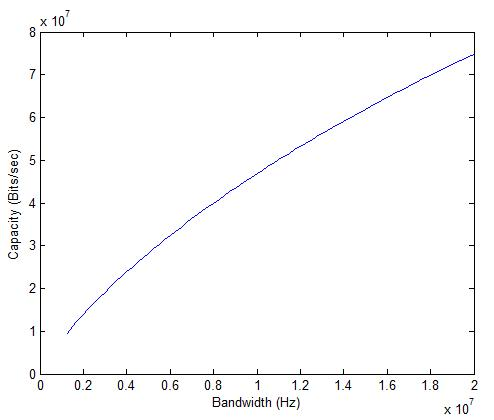# Category Archives: Channel Capacity

Maximum throughput as predicted by Shannon.

# Why is MIMO Fading Capacity Higher than AWGN Capacity

In a previous post we have seen that MIMO fading capacity is much higher than AWGN capacity with multiple antennas. How is this possible? How can randomness added by a fading channel help us? In this post we try to find the reason for this. Let’s assume the following signal model for a Multi Input Multi Output antenna system.

x=Hs+w

Here s is the NT by 1 signal vector, w is the NR by 1 noise vector and H is the NR by NT channel matrix. The received signal vector is represented by x which has dimensions of NR by 1. In expanded form this can be written as (assuming NT =4 and NR =4):

# Average Cell Throughput Calculations for LTE

Average Cell Throughput requires the following simulation results

• Average SINR distribution table (system level result), which provides the SINR probability

• Average throughput or spectral efficiency versus average SINR table (link level result)

For urban channel model and a fixed inter-site distance of 1732m,downlink throughput for LTE for different values of SINR is shown below.

Average Cell Throughput=Σ(Pi*Ri)

where

Pi=Probability of occurrence of a specific SINR value at cell edge obtained using simulations
Ri=Average throughput corresponding to SINR range

Let us consider the following distribution for the SINR at the cell edge:

P1=0.5 (SINR=1.50-3.50 dB)
P2=0.25 (SINR=3.50-7.00 dB)
P3=0.15 (SINR=7.00-9.50 dB)
P4=0.10 (SINR=9.50-11.50 dB)

Cell Throughput=(0.50*4)+(0.25*6)+(0.15*8)+(0.10*12)=5.9 Mbps

Note: This throughput is much less than the theoretical maximum which assumes that the full 20 MHz bandwidth is being utilized in a 4×4 MIMO configuration.

# Shannon Capacity of LTE (Ideal)

Shannon Capacity of LTE in AWGN can be calculated by using the Shannon Capacity formula:

C=B*log2(1+SNR)

or

C=B*log2(1+P/(B*No))

The signal power P is set at -90dBm, the Noise Power Spectral Density No is set at 4.04e-21 W/Hz (-174dBm/Hz) and the bandwidth is varied from 1.25MHz to 20MHz.It is seen that the capacity increases from about 10Mbps to above 70Mbps as the bandwidth is varied from 1.25MHz to 20MHz (keeping the signal power constant). It must be noted that this is the capacity with a single transmit and single receive antenna (MIMO capacity would obviously be higher).